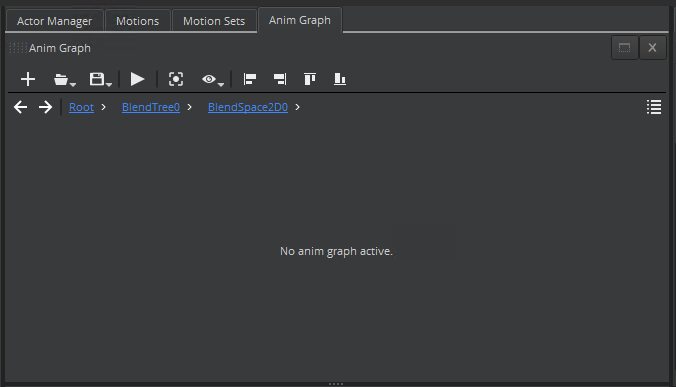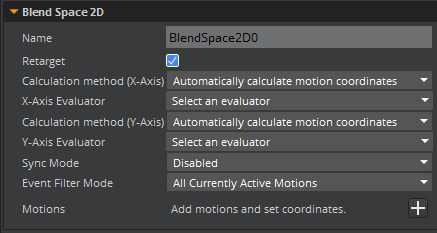Version:

# Creating and Visualizing Blend Spaces

Blend spaces are a collection of sample motions that are organized spatially according to their coordinates. The visual representation is a graph with xy-axes for the coordinates. The xy-axes can represent values such as move speed, travel direction, turn angle, and so on. Each motion is represented by a point (white dot) in the graph or blend space.

When you pick a point in the blend space (interactively or with parameter controls), your character automatically plays a resulting motion that is computed based on the sample motions and appropriate blend weights.

In a 1D blend space the motions correspond to points along a line. In a 2D blend space the motions correspond to points in a 2D space.

## Prerequisites

Before you can add blend space nodes to the animation graph, you must have completed the following:

• Selected an actor
• Selected a motion set
• Created an animation graph

## Creating Blend Spaces

To create a blend space, you must add a blend tree node and a blend space node, and then specify values for the attributes of the blend space node.

Blend space nodes output a pose from the Output Pose port. You can connect this output to the Input Pose port of the Final Output node or to the input port of any other node that accepts a pose as input.

The Blend Space 1D node has the following ports:

• X - The value for this input port indicates the current position of interest in the 1D blend space.
• Output Pose - The blend space node computes the blended motion that corresponds to the current position of interest and outputs the resulting motion from this port.

The Blend Space 2D node has the following ports:

• X - The value for this input port is the x-coordinate of the current position of interest in the 2D blend space.
• Y - The value for this input port is the y-coordinate of the current position of interest in the 2D blend space.
• Output Pose - The blend space node computes the blended motion that corresponds to the current position of interest and outputs the resulting motion from this port.

To create a blend space and specify attributes

1. In the Animation Editor, on the Anim Graph tab in the top middle pane, right-click the grid and then choose Create Node, Sources, Blend Tree.

2. Double-click the Blend Tree node to go to the blend tree view.

3. Add a blend space node to the blend tree by doing one of the following:

• On the Anim Graph tab, in the blend tree view, right-click the grid and then choose Create Node, Blending, Blend Space 2D or Blend Space 1D.
• In the Anim Graph Palette, on the Blending tab, drag the Blend Space 2D or Blend Space 1D icon into the blend tree view.
4. Double-click the blend space node to go to the blend space view. If you are using the Blend Space 2D node, your view should look as follows:1. In the Attributes pane, specify values for the attributes of the blend space node. These values are used to set up your blend space.

Note:
You can undock the Attributes pane in order to see the attributes and values without scrolling.• To use provided values for the xy-axes, do the following:

1. For Calculation method (X-Axis), select Automatically calculate motion coordinates.

2. For X-Axis Evaluator, select a common motion characteristic.

3. For Calculation method (Y-Axis), select Automatically calculate motion coordinates.

4. For Y-Axis Evaluator, select another common motion characteristic.

• To use custom values for the xy-axes, do the following:

1. For Calculation method (X-Axis), select Manually enter motion coordinates.

2. For Calculation method (Y-Axis), select Manually enter motion coordinates.

You can also use a combination of provided and custom values. For example, you can manually enter motion coordinates for the x-axis and automatically calculate motion coordinates for the y-axis using the Travel distance evaluator.

2. In the Attributes pane, for Motions, click the + button to add the source motion assets for your blend space.

3. In the Motion Selection Window, choose the motions that you want to add to the blend space, and then click OK.

• The coordinate value automatically calculates if you selected Automatically calculate motion coordinates for Calculation method and if you selected a motion characteristic for the Evaluator.
• You must enter coordinate values if you selected Manually enter motion coordinates for Calculation method.
4. After your motions are added to the blend space and the coordinate values are calculated, verify that your blend space view looks similar to the following:1. In the blend space view, do the following:

1. Drag within the blend space to change the point of interest (represented by a red dot).

2. When the point is highlighted, the corresponding motion is automatically computed by blending the motions represented by the three vertices of the triangle. View the blend weights next to each of the motions.

3. Notice that the motions that are closer to the point have a higher blend weight than the motions that are farther away.

2. In the Attributes pane, you can do the following:

• View the coordinate values for each motion
• Change the values to remap the animations and the blend space graph
• Remove a motion from the blend space